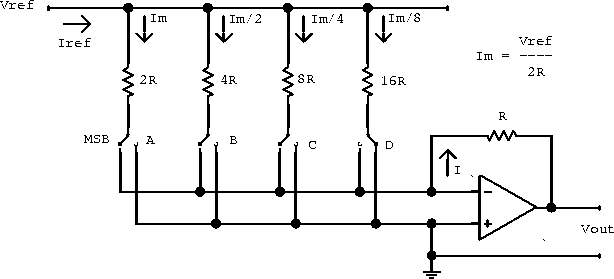Next: DAC Limitations Up: Digital-to-Analog Conversion Previous: Digital-to-Analog Conversion

## Current Summing and IC Devices

DACs are normally switched current devices designed to drive the current-summing junction of an operational amplifier. Figure 8.2 shows a simple 4-bit DAC.Figure 8.2:  The current DAC uses the summing input to an op-amp to yield a voltage output. LSB and MSB refer to the least and most significant bits of a binary number.

Each current is proportional to the value of a bit position in a binary number.

The design requires a number of different precisely defined resistor values. We can improve the circuit by replacing it with a circuit that requires fewer distinct resistor values.

Doug Gingrich
Tue Jul 13 16:55:15 EDT 1999